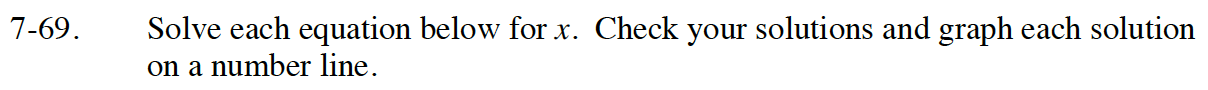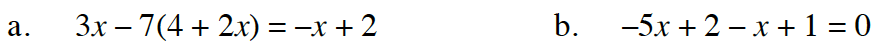Home > CC4 > Chapter 7 > Lesson 7.1.6 > Problem7-69

7-69.
1. Solve each equation below for x. Check your solutions and graph each solution on a number line. Homework Help ✎

1. 3x − 7(4 + 2x) = −x + 2

2. −5x + 2 − x + 1 = 0Read Math Notes box in Lesson A.1.8 on solving a linear equation and checking solutions.

x = −3